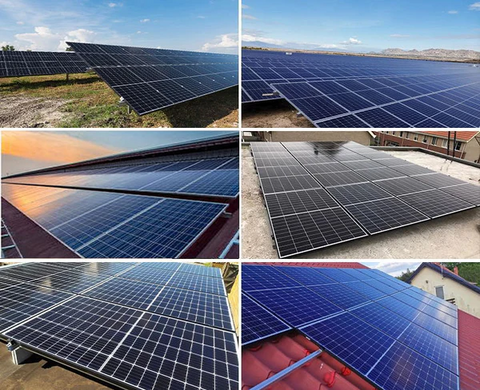# Do you know how many kilowatt-hours can a solar panel of 1 square meter generate in a day?With the rapid development of new energy technology, people's awareness of renewable energy has been continuously improved, and the use of solar panels has gradually entered the public's view. In the past decade, people have been asking passers-by in the street what solar energy may be regarded as an alien. Now more and more solar derivatives have entered into thousands of households, and the answer to the question may be eloquent and reasonable. The application of solar energy has a characteristic that it can be customized according to the environment. How much electricity can the solar panel generate in a day under the limit of one square meter?

If you want to know how much electricity a square meter of solar panels produce, you need to consider some major factors that affect their power generation efficiency. Light angle, light intensity, regional location, weather conditions, pollution, etc. will affect the power generation efficiency of solar panels. In order to avoid this situation, we can design a standard and conduct a theoretical calculation. At present, solar panels mostly use monocrystalline silicon and polycrystalline silicon, Its photoelectric conversion rate is not less than 15.5% - 16%. In the case of good weather at noon in summer, the solar radiation energy per square meter is about 1000W, and the average sunshine duration is about 6 hours. A solar panel of one square meter, under this standard condition, according to the formula of solar panel power conversion (length × wide × Conversion rate × 0.1=power) (unit: cm): 100 × one hundred × zero point one six × 0.1＝160W，160W × 6h=960Wh=0.96KWh is equal to 0.96 kWh. However, there must be errors in the actual situation. In reality, there must be various factors. Let's take these factors into account and calculate at 75% conversion rate, then we can get 0.96 × 75%=0.72 kWh.

In a word, considering the influence of various factors, after a theoretical calculation, it is concluded that a solar panel with the size of one square meter can generate about 0.72 degrees of electricity in a day.